## 5.14Descent methods

The Gauss-Seidel method, introduced in Sec. 5.2 , provides convergent solutions for many problems in CFD. It is most eﬀective when a modest reduction in residual is required, e.g. as part of a steady-state solution described in Sec. 5.12 .

When the Gauss-Seidel method requires a lot of sweeps (e.g. over 10) to converge to a suitable tolerance, alternative methods may be more eﬃcient. Descent methods provide alternative matrix solvers that are often used in CFD.

Descent methods represent the equations which are being solved as a minimisation problem. It is demonstrated below using a matrix equation of the form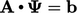with 2 values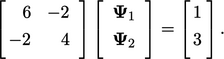(5.27)
The minimisation presents the equation in quadratic form; in matrix notation, it is a scalar function of the form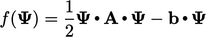(5.28)
The quadratic form of Eq. (5.27 ), is: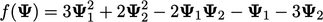(5.29)
The gradient of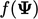is: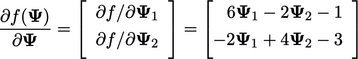(5.30)
Critically,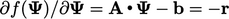, i.e. the negative of the residual vector Eq. (5.10 ), as veriﬁed by the model example.

Equating the gradient to zero,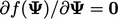, corresponds to a minimum in the quadratic function. At the same time, it is the solution to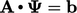. The method is therefore concerned with ﬁnding the minimum of the quadratic form eﬃciently.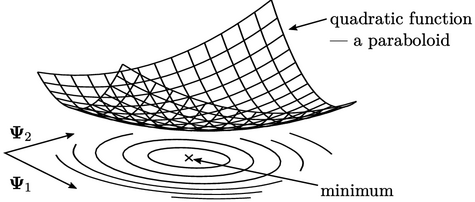For this method to work, the quadratic form must have a minimum, which requires that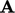is symmetric and positive-deﬁnite. A positive-deﬁnite matrix is hard to visualise, but for a 2-value function it ensures the quadratic function is a paraboloid.

Diagonal dominance is the convergence condition for the Gauss-Seidel method, discussed in Sec. 5.3 . Importantly, a symmetric matrix that is diagonally dominant, and has positive diagonal coeﬃcients, is also positive-deﬁnite.

### Matrix operations

The ‘’ operation between two single-column matrices, e.g.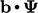, in Eq. (5.28 ) is represented in other texts using matrix notation by a transpose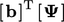. For the example in Eq. (5.27 ), it is: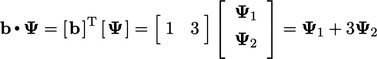(5.31)
Notes on CFD: General Principles - 5.14 Descent methods